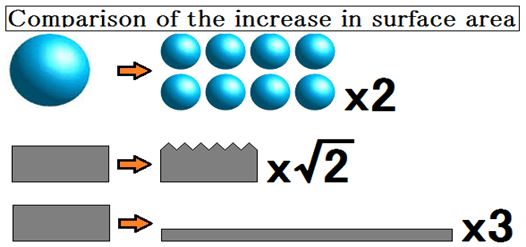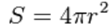# 4-4.Drying speed and swept area of the hot airUnit area (A) x Unit time (s) The amount of evaporation of moisture (em) is defined as Constant drying rate (Rc).
This means not being dependent on material and being dependent on the temperature, humidity, and speed of a hot air.
Because it is the product of the drying rate and surface area, if surface area is enlarged, an amount of evaporation will increase and a drying rate will increase.

To take a large surface area,
(1) Breaks finely.
(2) Attach wrinkles or hairline.
(3) Extend and stretched material.
There are methods such as.

The quantity of heat conveyed by convective heat transfer between the solid surface and fluid is called the heat transfer coefficient.
The heat transfer coefficient of a dry condition is proportional to the size of material.
Moreover, Moreover, it is in inverse proportion to √2 of volume.

### 4-4-1.The crushed mass of spherical, diameter is made into a half, divides into eight.

#### Surface areaS : surface area
π: Circular constant

In the sphere of the original, r = 1. therefore 4π
In the sphere of half the diameter, divided into 8 、r = 0.5. therefore 4πx0.5×0.5 =4πx0.25 =1π
However, since there are 8 pieces、1πx=8π

The crushed mass of spherical, diameter is made into a half, divides into eight.The surface area become 2 times.

#### Heat transfer coefficient

h ∝ √2V/1 ≒ 1.4times

h : Heat transfer coefficient
V : Volume

That is,
The crushed mass of spherical, diameter is made into a half, divides into eight.The surface area become 2 times.
Since the surface area at twice and the heat transfer coefficient 1.4 times,
Drying efficiency becomes better about 2.8 times.

### 4-4-2.Make the wrinkles on the surface of the solid

Make wrinkles jagged a 45-degree angle.Surface area will be √2 ≒ 1.4 times.

Therefore, the area become 1.4 times, and drying efficiency also increases 1.4 times.
In order to improve the drying efficiency, hair line finish is more advantageous than mirror surface finish.

### 4-4-3.Solid is rolled, and thickness is into one third.

Thickness become 1/3, surface area will increase 3 times.

Therefore, if area increases 3 times, drying efficiency will also increase 3 times.
In the case of a thin board, the heat transfer coefficient is also improved, and also drying efficiency becomes better.